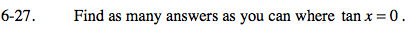### Home > PC > Chapter 6 > Lesson 6.1.2 > Problem6-27

6-27.

Find as many answers as you can where tan x = 0. Homework Help ✎$\tan(\theta) =\frac{\sin(\theta)}{\cos(\theta)}$

Tangent is zero when sin(θ) = 0.